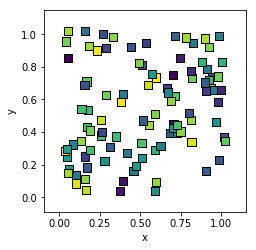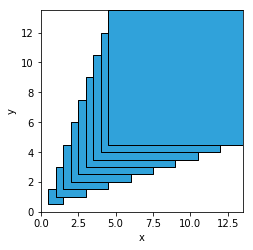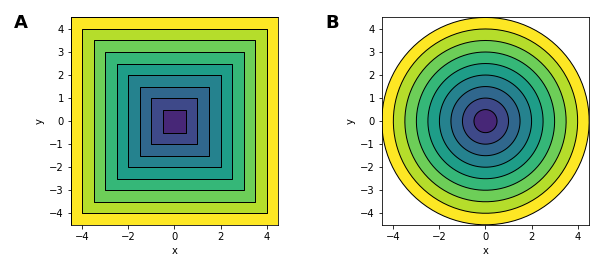# Polygons¶

Title
Polygons Element
Dependencies
Matplotlib
Backends
Matplotlib
Bokeh
In :
import numpy as np
import holoviews as hv
hv.extension('matplotlib')A Polygons represents a contiguous filled area in a 2D space as a list of polygon geometries. Just like the Contours element additional scalar value dimensions maybe may be supplied, which can be used to color the Polygons with the defined cmap. Like other Path types it accepts a list of arrays, dataframes, a dictionary of columns (or any of the other literal formats including tuples of columns and lists of tuples), but also supports a special 'holes' key to represent empty interior regions. For a full description of the polygon geometry data model see the Geometry Data User Guide.

In order to efficiently represent the scalar values associated with each path the dictionary format is preferable since it can store the scalar values without expanding them into a whole column. Additionally it allows passing multiple columns as a single array by specifying the dimension names as a tuple.

In this example we will create a list of random polygons each with an associated level value. Polygons will default to using the first value dimension as the color but for clarity we will define the color explicitly:

In :
def rectangle(x=0, y=0, width=.05, height=.05):
return np.array([(x,y), (x+width, y), (x+width, y+height), (x, y+height)])

polys = hv.Polygons([{('x', 'y'): rectangle(x, y), 'level': z}
for x, y, z in np.random.rand(100, 3)], vdims='level')


Out:Polygons is a very versatile element which may be used to draw custom annotations, choropleth maps (as can be seen in the texas_unemploment example) among many other examples. We can also use some of the other path based annotations to quickly generate polygons, including Box, Bounds and Ellipse elements. In the simple case we can simply pass a list of these elements:

In :
hv.Polygons([hv.Box(i, i, i) for i in range(10)])

Out:Alternatively we can use the array method to return the x/y-coordinates of the annotations and define additional z-values by declaring a dictionary:

In :
hv.Polygons([{('x', 'y'): hv.Box(0, 0, i).array(), 'z': i} for i in range(10)[::-1]], vdims='z') +\
hv.Polygons([{('x', 'y'): hv.Ellipse(0, 0, (i, i)).array(), 'z': i} for i in range(10)[::-1]], vdims='z')

Out:For full documentation and the available style and plot options, use hv.help(hv.Polygons).